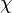# Polymer Solution Thermodynamics: Flory-Huggins Theory of Polymer Solutions

## Homework Statement

(Note: This isn't an assignment problem, more a curiosity about the derivation of an equation - hopefully it is still posted in the right forum..)

I have working through the derivation for the partial molar Gibbs free energy of mixing from the Flory-Huggins expression for the Gibbs free energy of mixing, however my math skills are (very) rusty - especially when it comes to partial derivatives.

## Homework Equations

Gibbs Free Energy of Mixing (Flory-Huggins Theory):
ΔGM=R·T[n1·ln(ϕ1)+n2·ln(ϕ2)+n1··ϕ2]

partial molar Gibbs free energy of dilution:
Δμ1=R·T[ln(1-ϕ2)+(1-1/r)·ϕ2 +·ϕ22]

where
KB=Boltzmann Constant,
ni=number of moles of i,
ϕi=volume fraction of i,=Flory-Huggins Interaction Parameter

ϕ1=N1/N0=N1/(N1+r·N2)
ϕ2=(r·N2)/N0=(r·N2)/(N1+r·N2)
N0=N1+r·N2

where
N0 is the number of lattice sites
N1 is the number of solvent molecules
N2 is the number of polymer molecules, each occupying "r" lattice sites (or "r" segments)

and R=KB·NA
where

## The Attempt at a Solution

ΔGM=R·T[n1·ln(ϕ1)+n2·ln(ϕ2)+n1··ϕ2]

applying R=KB·NA
ΔGM=KB·T[N1·ln(ϕ1)+N2·ln(ϕ2)+N1··ϕ2]

expressing ϕ1 and ϕ2 in terms of N1 and N2 gives
ΔGM=KB·T[N1·ln(N1/(N1+r·N2))+N2·ln((r·N2)/(N1+r·N2))+N1··(r·N2)/(N1+r·N2)]

Next step would be to take the partial derivative of the equation with respect to N1, I've tried many times but I cannot get anything close to the equation for the partial molar Gibbs free energy of dilution..

I broke it up, letting
Ψ1=N1·ln(N1/(N1+r·N2))
Ψ2=N2·ln((r·N2)/(N1+r·N2))
Ψ3=N1··(r·N2)/(N1+r·N2)

taking the partial derivative
1/dN1=ln(N1/(N1+rN2)+[1-(N1+rN1N2)/(N1+rN2)]
2/dN1=-N2[(1+rN2)/(N1+rN2)]
2/dN1=[(rN2)/(N1+rN2)]+N1[(-rN2)/(N1+rN2)2

which when I plug it all back in, gives me a big mess..

Last edited by a moderator:

Chestermiller
Mentor

## Homework Statement

(Note: This isn't an assignment problem, more a curiosity about the derivation of an equation - hopefully it is still posted in the right forum..)

I have working through the derivation for the partial molar Gibbs free energy of mixing from the Flory-Huggins expression for the Gibbs free energy of mixing, however my math skills are (very) rusty - especially when it comes to partial derivatives.

## Homework Equations

Gibbs Free Energy of Mixing (Flory-Huggins Theory):

partial molar Gibbs free energy of dilution:

where
KB=Boltzmann Constant,
ni=number of moles of i,
ϕi=volume fraction of i,

ϕ1=N1/N0=N1/(N1+r·N2)
ϕ2=(r·N2)/N0=(r·N2)/(N1+r·N2)
N0=N1+r·N2

where
N0 is the number of lattice sites
N1 is the number of solvent molecules
N2 is the number of polymer molecules, each occupying "r" lattice sites (or "r" segments)

and R=KB·NA
where

## The Attempt at a Solution

applying R=KB·NA

expressing ϕ1 and ϕ2 in terms of N1 and N2 gives

Next step would be to take the partial derivative of the equation with respect to N1, I've tried many times but I cannot get anything close to the equation for the partial molar Gibbs free energy of dilution..

I broke it up, letting
Ψ1=N1·ln(N1/(N1+r·N2))
Ψ2=N2·ln((r·N2)/(N1+r·N2))

taking the partial derivative
1/dN1=ln(N1/(N1+rN2)+[1-(N1+rN1N2)/(N1+rN2)]
2/dN1=-N2[(1+rN2)/(N1+rN2)]

which when I plug it all back in, gives me a big mess..
Start out with ##\frac{\partial \psi _3}{\partial n_1}##. You need to reduce the rhs to the least common denominator. You almost have it. At least get that term.

Chet

Last edited by a moderator:
•tetrakis
Start out with ##\frac{\partial \psi _3}{\partial n_1}##. You need to reduce the rhs to the least common denominator. You almost have it. At least get that term.

Chet

Hey Chet,
Thanks for the help, I've got the third term now. Am I even close with the other 2 terms? I've been playing around with them a bit more, but I can't quite seem to get it, especially the first term with the ln(1-ϕ2)

for ##\frac{\partial \psi _2}{\partial N_1}## I'm taking ##\frac{\partial N _2}{\partial N_1}## and ##\frac{\partial ln(rN_2)}{\partial N_1}## as zero based on the assumption that it is similar to taking the derivative of a constant, would this be correct?

Chestermiller
Mentor
for ##\frac{\partial \psi _2}{\partial N_1}## I'm taking ##\frac{\partial N _2}{\partial N_1}## and ##\frac{\partial ln(rN_2)}{\partial N_1}## as zero based on the assumption that it is similar to taking the derivative of a constant, would this be correct?
This is correct. But your partial derivatives of psi1 and psi2 with respect to n1 are both incorrect. You need to be more careful. Try again.

Also note the phi1 = 1 - phi2

Chet

This is correct. But your partial derivatives of psi1 and psi2 with respect to n1 are both incorrect. You need to be more careful. Try again.

Also note the phi1 = 1 - phi2

Chet
Thank you, I've gotten the correct equation now.